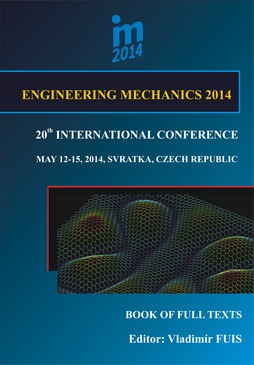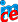# Engineering Mechanics

## International Conference

### Proceedings Vol. 20 (2014)### ENGINEERING MECHANICS 2014

20th INTERNATIONAL CONFERENCE
May 12 – 15, 2014, Svratka, Czech Republic
;

Copyright © 2014 Brno University of Technology Institute of Solid Mechanics, Mechatronics and Biomechanics

ISBN 978-80-214-4871-1 (printed)
ISSN 1805-8248 (printed)
ISSN 1805-8256 (electronic)

list of papers scientific commitee

PARAMETER IDENTIFICATION IN INITIAL VALUE PROBLEMS FOR NONLINEAR ORDINARY DIFFERENTIAL EQUATIONS

Nonlinear initial value problems (IVPs) for ordinary differential equations are considered. As a representative, a cement hydration model is chosen. The model equation depends on a few parameters that are to be identified on the basis of hydration-related measurements at a sequence of time points. This is done through the minimization of a cost function defined as the sum of squared differences between the measured values and the model response at the same time points. To minimize the cost function, a gradient based algorithm is used. The gradient of the cost function can be calculated either by numerical differentiation or via solving auxiliary initial value problems. The minimization algorithm tends to find a local minimum. Therefore, it is run from different starting points to increase the chance of finding the global minimum. Algorithms are coded in the Matlab environment, and Matlab IVP solvers as well as Matlab Optimization Toolbox and Symbolic Math Toolbox are utilized. The latter makes the derivation of the auxiliary IVPs easy and reliable.

back to list of papers

Text and facts may be copied and used freely, but credit should be given to these Proceedings.

All papers were reviewed by members of the scientific committee.Powered by Imce 3.12  © 2019, Pavel Formánek, Institute of Thermomechanics AS CR, v.v.i. [generated: 0.0218s]